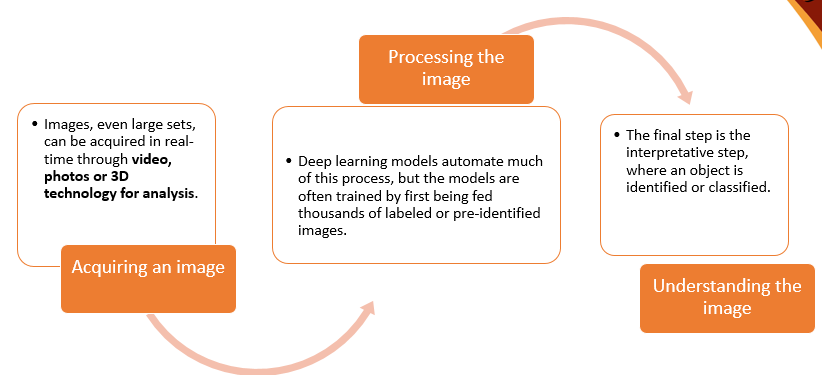# An Introduction to OpenCV using  Google Colab Notebooks- Part 1

Original article was published by Reny Jose on Artificial Intelligence on MediumOpenCV stands for Open Source Computer Vision library and was invented by Intel in 1999. It is used for image processing and computer vision. Computer vision is a subfield of artificial intelligence where we train computers to interpret and understand the visual world.

How computer vision works

Import OpenCV

Before doing that we need to mount the google drive

Using the above code I mount my drive the default path is ‘/content/drive’. To set the path or working folder as OPENCV, I used os.chdir and sys.path.append. So now my current folder is ‘/content/drive/My Drive/Colab Notebooks/OPENCV’.

To read, write and display an image

Using the above code we can read an image with cv2.imread, cv2_imshow to display the image, and cv2.imwrite to write the image drive.

To know the size, reshape, copy, etc

If the image is grayscale size contains only width and height. But if it is a color image the output contains the width, height, and channel. fig 4.we can read an image as grayscale bypassing the value 0 and BGR as bypassing value 1 in imread command.

To resize an image we pass the width and height as a tuple. here I resize the image to (400,300) size.

To copy an image we use the command image.copy() command. here in code ‘img’ is a variable that stores an image by using the command imread.

# Basic geometric Operations

The basic geometric operations are

1. Create a black and white image

By using the NumPy package we can create an image. First, we are importing the package, and using that we pass the size of the image as a tuple(width, height) and datatype as type. We can also use matplot lib to display the image. for that first ‘import matplotlib.pyplot as plt’ and the use the command plt.imshow().

2. Create a black and white image

The only difference is when we pass the size of the image as a tuple(width, height, channel) where the channel is 3 for BGR types of image.

3. Draw line

To draw a line we use the command line which takes the parameters (image, the starting point as a tuple, ending point as a tuple, color values(R, G, B), the thickness of the line). If you want to get different color combinations just go to the google and type RGB color picker you will get the different color values.

4. Draw Arrowed Line

To draw an arrowed line we use the command arrowedLine with the same parameter as the line.

5. Draw Rectangle

To draw a rectangle we use the command rectangle( image, top left point as a tuple, bottom right point as a tuple, color(R, G, B), thickness, ) also we can specify the line types like doted, etc). If the thickness value is -1 it will fill the shape with color.

6. Draw Circle

To draw a circle we use command cv2.circle with parameter (image, center_coordinates as a tuple, radius, color(R, G, B), thickness)

7. Draw Ellipse

To draw an Ellipse we use command cv2.ellipse with parameter( image, center as a tuple, axes, angle, startAngle, entangle, color(R, G, B), thickness). Here angle containing the major and minor axis of the ellipse (major axis length, minor axis length). Both the startAngle, entangle are provides in degree.

8. Draw Polyline

To draw polyline we use the command cv2.polylines with parameters (image, points as an array, is closed which take True or False values, color(R, G, B), thickness)

9.Draw text on the image

To put text on an image we use the command putText with the parameters ( image, text, starting point as a tuple, font face, font scale, color (R, G, B), thickness, line type)

10 Crop the image

To crop the image we pass the starting point and ending points.

References

1. https://docs.opencv.org/master/dc/da5/tutorial_py_drawing_functions.html
2. Learning OpenCv2 Computer vision with Python by Joe Minichino and Joseph Howse
3. OpenCV with python by examples by Prateek Joshi